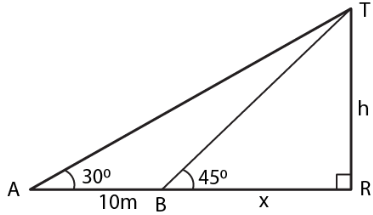Guru

# The shadow of a vertical tower on a level ground increases by 10 m when the altitude of the sun changes from 450 to 300. Find the height of the tower, correct to two decimal places.

• 0

sir this is the question from the book -ML aggarwal( avichal publication) class 10th , chapter20 , heights and distances
wehave the information that The shadow of a vertical tower on a level ground increases by 10 m
when the altitude of the sun changes from 450 to 300.
wehave to Find the height of the tower, correct to two decimal places. please solve this providing figure
question no 18 , heights and distances , ICSE board

Share

1. In the figure

AB is the tower

BD and BC are the shadow of the tower in two situations

Consider BD = x m and AB = h mIn triangle ABD

tan 450 = h/x

So we get

1 = h/x

h = x ….. (1)

In triangle ABC

tan 300 = h/(x + 10)

So we get

1/√3 = h/(x + 10)

Using equation (1)

h√3 = h + 10

h (√3 – 1) = 10

We know that

h = 10/(√3 – 1)

It can be written as

h = [10 (√3 + 1)]/ [(√3 – 1) (√3 + 1)]

By further calculation

h = (10√3 + 1)/ 2

So we get

h = 5 (1.73 + 1)

h = 5 × 2.73

h = 13.65 m

Therefore, the height of the tower is 13.65 m.

• 0